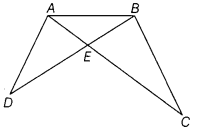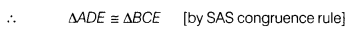# In the given figure, ∠EBA = ∠EAB and AC = BD. Prove that AD = BC

In the given figure, ∠EBA = ∠EAB and AC = BD. Prove that AD = BCGiven, ∠EBA =∠EAB
∴ AE = BE
[sides oppsite to equal angles are equal]
and AC = BD (given)
=>AE + EC = BE + ED
=>EC=ED[AE=BE]